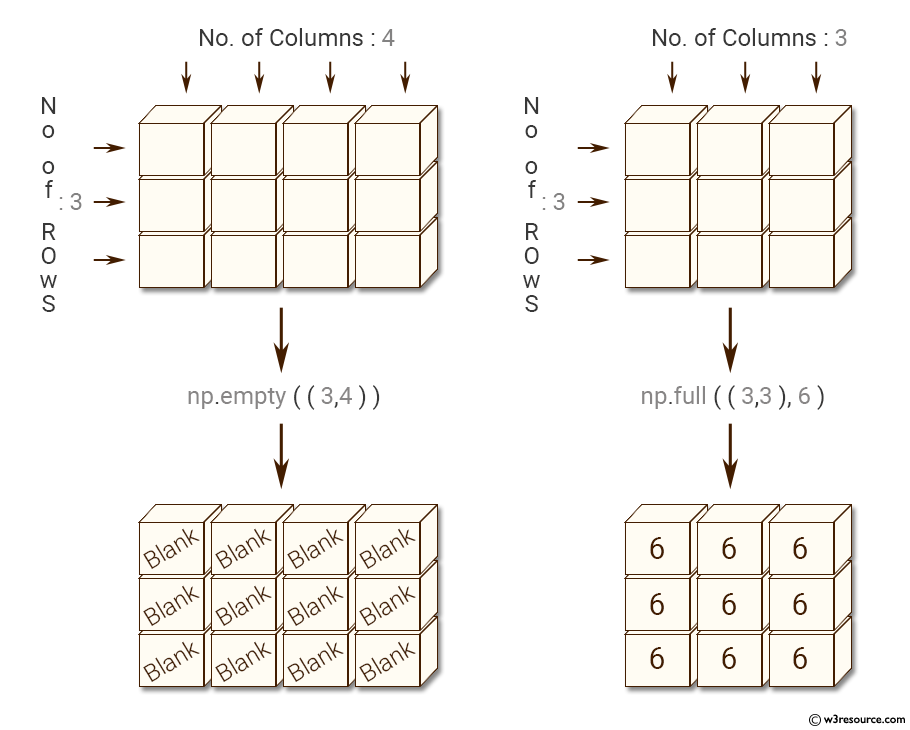﻿ NumPy: Create an empty and a full array - w3resource# NumPy: Create an empty and a full array

## NumPy: Array Object Exercise-13 with Solution

Write a NumPy program to create an empty and a full array.Sample Solution:-

Python Code:

``````import numpy as np
# Create an empty array
x = np.empty((3,4))
print(x)
# Create a full array
y = np.full((3,3),6)
print(y)
```
```

Sample Output:

```[[  6.93643969e-310   8.76783124e-317   6.93643881e-310   6.79038654e-31
3]
[  2.22809558e-312   2.14321575e-312   2.35541533e-312   2.42092166e-32
2]
[  7.46824097e-317   9.08479214e-317   2.46151512e-312   2.41907520e-31
2]]
[[6 6 6]
[6 6 6]
[6 6 6]]
```

Python Code Editor:

Have another way to solve this solution? Contribute your code (and comments) through Disqus.

What is the difficulty level of this exercise?

Test your Python skills with w3resource's quiz

﻿

## Python: Tips of the Day

Set comprehension:

```>>> m = {x ** 2 for x in range(5)}
>>> m
{0, 1, 4, 9, 16}
```